# On the Numerical Treatment of Magneto-Hydro Dynamics Free Convection with Mixed Boundary Conditions

On the Numerical Treatment of Magneto-Hydro Dynamics Free Convection with Mixed Boundary Conditions
Laboratory of Thermal and Energetic Systems Studies (LESTE) at the National School of Engineering of Monastir, University of Monastir, Monastir 5000, Tunisia

Preparatory Institute of Engineering Studies of Monastir (IPEIM), University of Monastir, Monastir 5000, Tunisia

Corresponding Author Email:
raoudhach@gmail.com
Page:
421-426
|
DOI:
https://doi.org/10.18280/mmep.070312
24 August 2018
|
Accepted:
12 March 2020
|
Published:
30 September 2020
| Citation

OPEN ACCESS

Abstract:

In this paper, Lattice Boltzmann method (LBM) is proposed to simulate Magneto-hydrodynamic (MHD) free convection in a two-dimensional open cavity with mixed boundary conditions (BCs). The cavity is getting under a uniform transverse magnetic field. The proposed numerical scheme solved the flow field and the temperature field using D2Q9 lattice model. So, the main aim of this study is to highlight the effectiveness of this mesoscopic model to predict the effects of pertinent parameters such as the Hartmann number varying from 0 to 150 and the Prandtl number altering in a wide range of Pr=0.025 and 0.71. Rayleigh number is fixed at moderate value of 105. This in-house numerical code used in this paper is ascertained and a good agreement with literature is highlighted. The appropriate validation with previous numerical investigations demonstrated that this attitude is a suitable method and a powerful approach for engineering MHD problems. Findings and results show the alterations of Hartman number that influence the isotherms and the streamlines widely at different Rayleigh and Prandtl numbers simultaneously. Moreover, heat transfer declines with the increment of Hartmann number. The effect of the magnetic field on the average Nusselt number at Liquid Gallium (Pr=0.025) is also highlighted.

Keywords:

mixed BC, convection, heat transfer, LBM linearly, MHD, open cavity, convection, linearly, heat transfer

1. Introduction

Free convection in closed and open cavities has many engineering applications such as: cooling systems of solar collectors, electronic components, building and thermal insulation systems, nuclear reactor systems, food storage industry and geophysical fluid mechanics. Convection under the influence of a magnetic field received a considerable attention in crystal growth in fluids, metal casting, fusion reactors and geothermal energy extractions, natural convection is under the influence of a magnetic field [1-20]. Recent attention has been intensively focused on the cases with mixed boundary conditions on the walls of an open cavity [21-24].

Over the last decades, LBM was an applicable method for simulating fluid flow and heat transfer successfully [25-32]. It becomes a powerful, an effective and easy numerical method, it is used in simulating complex flow problems with different boundary conditions [33, 34].

This surge in interest of the D2Q9 LBM (Figure 1-c) model is mainly attributed to its computational simplicity, direct discretization, ability and efficiency. It is known that the conventional computational fluid dynamics (CCFD) solvers, namely the volume finite element method (FVM), the control volume finite element method (CVFEM), the finite difference method (FDM) are macroscopic models but the LBM is a recent mesoscopic approach describing and capturing engineering physics better . This mesoscopic approach includes simple calculations procedure, efficient implementation for a parallel architecture and robustness for handling complex geometry

Engineering applications including linear, sinusoidal, convective, Dirichlet, open, Neumann Boundary Conditions (BC), or a mixture  of these conditions in different walls of the MHD cavity is a tricky task for numerical heat and mass transfer in physical engineering simulations.

This is a typical problem with mixed boundary conditions and should not be confused with the considerably simpler problem when the temperature is prescribed over certain complete sides of the rectangle, while the temperature gradient is prescribed over the remaining sides. As far as the writer is aware no analytical solution of the mixed boundary value problem above formulated (or of the analogous problem for the cylinder) is to be found in the literature. We must therefore (if interested in numerical answers) resort to the alternative of substituting for the differential equation of heat conduction and for the equations expressing the initial and boundary conditions their appropriate difference analogs, and solving the resulting system.

The main aim of this paper is to identify the ability of Lattice Boltzmann Method (LBM) for solving magnetic field simultaneously in the presence of a non isothermal boundary condition. It is endeavored to express the best situation for heat transfer and fluid flow with the MHD and mixed BCs parameters. The effects of Rayleigh number on streamlines, isotherms and the Nusselt number are investigated.

2. Mathematical Formulation

Plotting of considered model is shown in Figure 1. It displays a two-dimensional open cavity with side length of H. At first case the left vertical is maintained at high temperature (TH). Whereas at the second case, the vertical left wall is linearly heated. An external cold air enters into the enclosure from the east opening boundary, while the fluid is correlated with the opening boundary at constant temperature (TC). The horizontal walls are insulated and impermeable to mass transfer. The open cavity is filled with liquid gallium with Prandtl number of 0.025. The gravitational acceleration acts downward. The uniform external magnetic field with a constant magnitude B is applied in the x-direction (transverse field). It is assumed that the induced magnetic field produced by the motion of an electrically conducting fluid is negligible compared to the applied magnetic field. Thermo-physical properties of the fluid are assumed to be constant, and the density variation in the buoyancy force term is handled by the Boussinesq approximation. The flow is two-dimensional, laminar and incompressible; in addition, it is assumed that the viscous dissipation and Joule heating are neglected.

An open cavity is considered for the present study with the physical dimensions as shown in Figure 1. The left vertical is linearly heated. An external cold air enters into the enclosure from the east opening boundary, while the fluid is correlated with the opening boundary at constant temperature (Tc). The horizontal walls are insulated and impermeable to mass transfer. The open cavity is filled with liquid gallium. The gravitational acceleration acts downward.

## 1.pngFigure 1. Geometries (a) and D2Q9 velocities (b)

The uniform external magnetic field with a constant magnitude B0 is applied in the x-direction. The density variation in the fluid is approximated by the standard Boussinesq model. It is assumed that the induced magnetic field produced by the motion of an electrically conducting fluid is negligible compared to the applied one. Furthermore, it is assumed that Joule heating and the viscous dissipation are neglected. Therefore, standard D2Q9 for flow and for temperature can be written in non dimensional form as follows:

$\frac{\partial u}{\partial x}+\frac{\partial v}{\partial y}=0$            (1)

\begin{align} & \rho (\frac{\partial u}{\partial t}+u\frac{\partial u}{\partial x}+v\frac{\partial u}{\partial y})=-\frac{\partial p}{\partial x}+\mu (\frac{{{\partial }^{2}}u}{\partial {{x}^{2}}}+\frac{{{\partial }^{2}}u}{\partial {{y}^{2}}}) +\frac{H{{a}^{2}}\mu }{{{H}^{2}}}(v\sin \gamma \cos \gamma -u{{\sin }^{2}}\gamma ) \\ \end{align}                     (2)

\begin{align} & \rho (\frac{\partial v}{\partial t}+u\frac{\partial v}{\partial x}+v\frac{\partial v}{\partial y})=-\frac{\partial p}{\partial y}+\mu (\frac{{{\partial }^{2}}v}{\partial {{x}^{2}}}+\frac{{{\partial }^{2}}v}{\partial {{y}^{2}}}) +\frac{H{{a}^{2}}\mu }{{{H}^{2}}}(u\sin \gamma \cos \gamma -v{{\cos }^{2}}\gamma )+\rho g\beta (T-{{T}_{m}}) \\ \end{align}               (3)

$\frac{\partial T}{\partial t}+u\frac{\partial T}{\partial x}+v\frac{\partial T}{\partial y}=\alpha (\frac{{{\partial }^{2}}T}{\partial {{x}^{2}}}+\frac{{{\partial }^{2}}T}{\partial {{y}^{2}}})$               (4)

Hartmann number is written as $H a=H B \sqrt{\sigma / \mu}$.

The macroscopic velocity and temperature are [25-34]:

$\text{u}(\text{r},t)=\sum\limits_{k}^{{}}{{{e}_{k}}{{f}_{k}}}(\text{r},t)/\sum\limits_{k}^{{}}{{{f}_{k}}(\text{r},t)}$                (5)

$T=\sum\limits_{k}^{{}}{{{g}_{k}}(\text{r},t)}$         (6)

Nusselt number Nu is one of the most important dimensionless parameters in describing the convective heat transport. The local Nusselt number and the average value at the bottom wall are calculated as;

$N{{U}_{y}}=-\frac{H}{\Delta T}\frac{\partial T}{\partial x}$                (7)

$N{{U}_{avg}}=\frac{1}{H}\int\limits_{0}^{H}{N{{U}_{y}}dy}$                (8)

3. Results and Discussions

In order to check the accuracy of the present results, the present code is validated against published works in the literature, in Figure 2 we compare the steady state isotherms at Pr=0.71 for Ra=105 in the absence of a magnetic field (Hartmann number, Ha=0) with reference , a good agreement is observed. A second validation is highlighted, for a different Prandtl number of Pr=0.025 (liquid gallium), the steady state isotherms of linearly heated side walls MHD cavity with a moderate Hartmann number of 50, Pr=0.025 and Ra=105. The obtained numerous investigations have been compared with reference  and present work. After grid assessment and validation with previous literature (Figure 2), we illustrate the effect of magnetic field in an open cavity with linearly heated west Boundary which is filled with liquid gallium for Ha=50 and Ra=105.

We seek to provide the behavior of the Nusselt bottom wall Nub in the case of two linear vertical walls. Figure 3 shows clearly that the local Nusselt number at the bottom wall Nub exhibits an oscillatory behavior with the horizontal distance x/X and that it is exactly symmetric about the centerline of the bottom wall. The Variation of local Nusselt number with distance at bottom wall in the case of linearly heated side walls (Pr= 0.025 and Ra=105) is depicted for different Hartman numbers.

In Figure 4 (a-b), the outlet section of flow on the open boundary moves downward and the movement of the flow gets limited with the increment of Hartmann number that it can influences heat transfer from the linearly heated wall to the cold open boundary. The effect of the presence of the magnetic field is clear in the counter of the isotherms where the isotherms recede from the linearly heated left wall slowly and their gradient on the left wall declines extremely which it exposes the decrease in heat transfer in the open cavity. Figure 4c highlight the variation of local Nusselt number Nub with horizontal distance X at bottom wall in the case of linearly heated side wall for a MHD open cavity (Pr=0.025 and Ra=105). We notice an increasing trend when the position vary from to 0 to left (west)-edge of the bottom side wall because of the linearly heated left boundary. Nub reach the maximum at the right (east)side of the bottom edge due to the cooled right (east) wall.

## 3a.png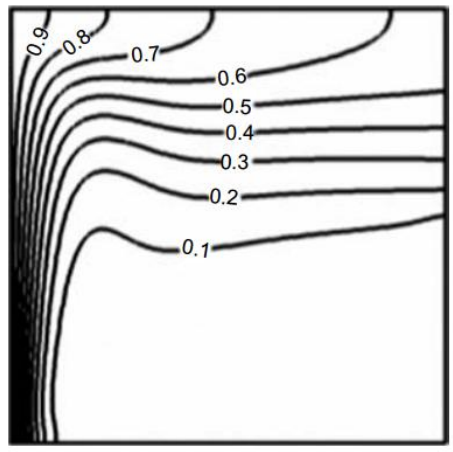(a)

## 3b.png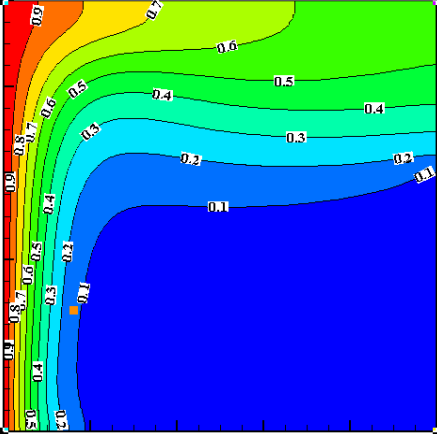(b)

## 3c.png(c)

## 3d.png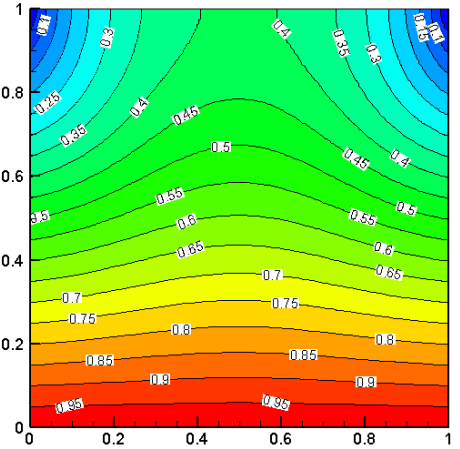(d)

Figure 2. (a-b) Comparison of the steady state isotherms (a-b) at Pr=0.71 for Ha = 0 and Ra = 105 (a) ref.  and (b) and (c-d) steady state isotherms of linearly heated side walls MHD cavity for Ha = 50, Pr=0.025 and Ra = 105, (c) ref.  and (d) present work

## 3.png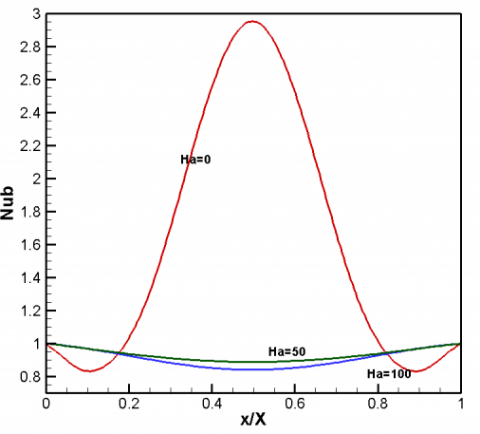Figure 3. Variation of local Nusselt number with distance at bottom wall in the case of linearly heated side walls (Pr=0.025 and Ra = 105)

## 4a.png(a)

## 4b.png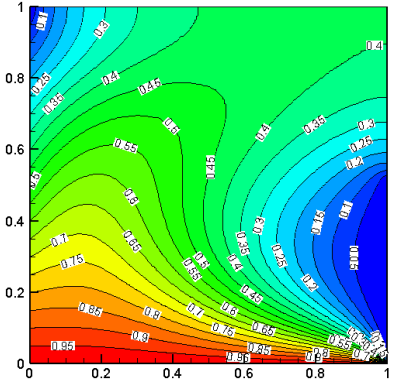(b)

## 4c.png(c)

Figure 4. Isotherms (a) and streamlines (b) and Variation of local Nusselt number (c) with distance at bottom wall in the case of linearly heated side wall for a MHD open cavity

4. Conclusions

In this work, a lattice Boltzmann method is proposed to simulate MHD natural convection of two-dimensional open square cavity with a linearly heated boundary condition. A D2Q9 lattice model is used both to simulate the flow field and temperature field. Aiming to validate the proposed model, the obtained results of this study have been compared with previous numerical investigations [7, 20]. It was shown that the results predicted by the proposed method are in good agreement with other numerical results. The obtained numerical results show that the LB model is a stable, powerful approach for simulating the MHD free convection in a two-dimensional open square cavity with mixed boundary conditions and is able to study the effects of all parameter on the flow field and temperature field such as Hartmann number and Prandtl number. Besides, the implementation of the new model highlights a great ability and stability. It is noted that, the application of the magnetic field reduces the convective heat transfer rate in the open enclosure. The profiles of the local Nusselt number along the bottom wall of the MHD enclosure increased continuously for the case of linearly heated left side wall and cooled open right wall, while it exhibited an oscillatory behavior along the horizontal distance for the case of linearly heated side walls. All simulations are done for Ra=105 when the buoyancy-driven flow starts to dominate the heat transfer mechanism.

Nomenclature
 Ha Hartmann number B magnetic field intensity, T ρ Density,kgm-3 u,v Velocities, ms-1 g k gravitational acceleration, m.s-2 thermal conductivity, W.m-1. K-1 Ra Rayleigh number Pr Prandtl number H characteristic length scale, m ei discrete particle speeds, ms-1 $\Delta T$ Temperature difference, k f density distribution functions g internal energy distribution functions T Temperature, k γ Angle, rad Nu local Nusselt number x,y cartesian coordinates, m Tm Avearge temperature, k Greek symbol a thermal diffusivity, m2. s-1 b thermal expansion coefficient, k-1 τ Relaxation time, s $\sigma$ electrical conductivity, Sm−1 µ dynamic viscosity, kg. m-1.s-1 ν kinematic viscosity, m2s-1 Subscripts avg average H Hot C Cold
References

 Polat, O., Bilgen, E. (2002). Laminar natural convection in inclined open shallow cavities. International Journal of Thermal Sciences, 4: 360-368. https://doi.org/10.1016/s1290-0729(02)01326-1

 Mohamad, AA. (1995). Natural convection in open cavities and slots. Numerial Heat Transfer, Part A: Applications, 27(6): 705-716. https://doi.org/10.1080/10407789508913727

 Mohamad, A.A., El-Ganaoui, M., Bennacer, R. (2009). Lattice Boltzmann simulation of natural convection in an open ended cavity. International Journal of Thermal Sciences, 48(10): 1870-1875. https://doi.org/10.1016/j.ijthermalsci.2009.02.004

 Mohamad, A.A., Bennacer, R., El-Ganaoui, M. (2010). Double dispersion natural convection in an open end cavity simulation via Lattice Boltzmann Method. International Journal of Thermal Sciences, 49(10): 1944-1953. https://doi.org/10.1016/j.ijthermalsci.2010.05.022

 Kennedy, P, Zheng, R. (2013). Flow Analysis of Injection Molds, Hanser, Munic.

 Al-Rashed, A., Kolsi, L., Kalidasan, K., Malekshah, E.H., Borjini, M.N., Kanna, P.R (2017). Second law analysis of natural convection in a CNT-water nanofluid filled inclined 3D Cavity with incorporated Ahmed Body. International Journal of Mechanical Sciences, 130: 399-415. https://doi.org/10.1016/j.ijmecsci.2017.06.028

 Sathiyamoorthy, M., Chamkha, A. (2010). Effect of magnetic field on natural convection flow in a liquid gallium filled square cavity for linearly heated side wall(s). International Journal of Thermal Sciences, 49(9): 1856-1865. https://doi.org/10.1016/j.ijthermalsci.2010.04.014

 Alchaar, S., Vasseur, P., Bilgen, E. (1995). Natural convection heat transfer in a rectangular enclosure with a transverse magnetic field. Journal of Heat Transfer, 117(3): 668-673. https://doi.org/10.1115/1.2822628

 Garandet, J., Alboussiere, T., Moreau, R. (1992). Buoyancy drive convection in a rectangular enclosure with a transverse magnetic field. International Journal of Heat and Mass Transfer, 35(4): 741-748. https://doi.org/10.1016/0017-9310(92)90242-K

 Rudraiah, N., Barron, R., Venkatachalappa, M., Subbaraya, C. (1995). Effect of a magnetic field on free convection in a rectangular enclosure. International Journal of Engineering Science, 33(8): 1075-1084. https://doi.org/10.1016/0020-7225(94)00120-9

 Cowley, M. (1996). Natural convection in rectangular enclosures of arbitrary orientation with magnetic field vertical. Magnetohydrodynamics, 32(4): 390-398. http://doi.org/10.22364/mhd

 Teamah, M.A. (2006). Hydro-magnetic double-diffusive natural convection in a rectangular enclosure with imposing an inner heat source or sink. Alex. Eng. J., 45(4): 401-415.

 Ozoe, H., Okada, K. (1989). The effect of the direction of the external magnetic field on the three dimensional natural convection in a cubic enclosure. Int. J. Heat Mass Transf, 32: 1939-1953.

 Ece, M., Buyuk, E. (2006). Natural convection flow under a magnetic field in an inclined rectangular enclosure heated and cooled on adjacent walls. Fluid Dynamics Research, 38(8): 546-590. https://doi.org/10.1016/j.fluiddyn.2006.04.002

 Al-Najem, N., Khanafer, K., El-Refaee, M. (1998). Numerical study of laminar natural convection in tilted enclosure with transverse magnetic field. International Journal of Numerical Methods for Heat & Fluid Flow, 8(6): 651-672. https://doi.org/10.1108/09615539810226094

 Jalil, J.M., Al-Taey, K.A. (2007). MHD turbulent natural convection in a liquid metal filed square enclosure. Emirates Journal for Engineering Research, 12(2): 31-40.

 Gelfgat, A., Bar-Yoseph, P. (2001). The effect of an external magnetic field on oscillatory instability of convective flows in a rectangular cavity. Physics of Fluids, 13(8): 2269-2278. https://doi.org/10.1063/1.1383789

 Aleksandrova, S., Molokov, S. (2004). Three-dimensional buoyant convection in a rectangular cavity with differentially heated walls in a strong magnetic field. Fluid Dynamics Research, 35(1): 37-66. https://doi.org/10.1016/j.fluiddyn.2004.04.002

 Kahveci, K., Oztuna, S. (2009). MHD natural convection flow and heat transfer in a laterally heated partitioned enclosure. European Journal of Mechanics-B/Fluids, 28(6): 744-752. https://doi.org/10.1016/j.euromechflu.2009.07.001

 Kefayati, G.H.R., Gorji, M., Ganji, D.D., Sajjadi, H. (2013). Investigation of Prandtl number effect on natural convection MHD in an open cavity by Lattice Boltzmann Method. Engineering Computations, 30(1): 97-116. https://doi.org/10.1108/02644401311286035

 Bilgen, E., Yedder, R.B. (2007). Natural convection in enclosure with heating and cooling by sinusoidal temperature profiles on one side. International Journal of Heat and Mass Transfer, 50(1-2): 139-150. https://doi.org/10.1016/j.ijheatmasstransfer.2006.06.027

 Sarris, I.E., Lekakis, I., Vlachos, N.S. (2002). Natural convection in a 2D enclosure with sinusoidal upper wall temperature. Numerical Heat Transfer, Part A: Application, 42(5): 513-530. https://doi.org/10.1080/10407780290059675

 Varol, Y., Oztop, H.F., Pop, I. (2008). Numerical analysis of natural convection for a porous rectangular enclosure with sinusoidally varying temperature profile on the bottom wall. International Communications in Heat and Mass Transfer, 35(1): 56-64. https://doi.org/10.1016/j.icheatmasstransfer.2007.05.015

 Saeid, N.H., Yaacob, Y. (2006). Natural convection in a square cavity with spatial sidewall temperature variation. Numerical Heat Transfer Application, 49(7): 683-697. https://doi.org/10.1080/10407780500359943

 Martinez, D., Chen, S., Matthaeus, W. (1994). Lattice Boltzmann magneto hydrodynamics. Physics of Plasmas, 1(6): 1850-1867. https://doi.org/10.1063/1.870640

 Chaabane, R., Askri, F., Ben Nasrallah, S. (2011). Parametric study of simultaneous transient conduction and radiation in a two-dimensional participating medium. Communications in Nonlinear Science and Numerical Simulation, 16(10): 4006-4020. https://doi.org/10.1016/j.cnsns.2011.02.027

 Saha, L.K., Hossain, M.A., Gorla, R.S.R. (2007). Effect of Hall current on the MHD laminar natural convection flow from a vertical permeable flat plate with uniform surface temperature. International Journal of Thermal Science, 46(8): 790-801. https://doi.org/10.1016/j.ijthermalsci.2006.10.009

 Chaabane, R., Askri, F., Ben Nasrallah, S. (2011). Analysis of two-dimensional transient conduction-radiation problems in an anisotropically scattering participating enclosure using the lattice Boltzmann method and the control volume finite element method. Journal of Computer Physics Communications, 182(7): 1402-1413. https://doi.org/10.1016/j.cpc.2011.03.006

 Lamsaadi, M., Naimi, M., Hasnaoui, M., Mamou, M. (2006). Natural convection in a vertical rectangular cavity filled with a non-Newtonian power law fluid and subjected to a horizontal temperature gradient. Numerical Heat Transfer, Part A: Application, 49: 969-990. https://doi.org/10.1080/10407780500324988

 Chaabane, R., Askri, F., Ben Nasrallah, S. (2011). Application of the lattice Boltzmann method to transient conduction and radiation heat transfer in cylindrical media. Journal of Quantitative Spectroscopy and Radiative Transfer, 112(12): 2013-2027. https://doi.org/10.1016/j.jqsrt.2011.04.002

 Chaabane, R., Askri, F., Jemni, A., Ben Nasrallah, S. (2017). Numerical study of transient convection with volumetric radiation using a hybrid lattice Boltzmann BGK-control volume finite element method. Journal of Heat Transfer, 139(9): 092701-092701-72017. https://doi.org/10.2298/TSCI100722015C

 Chaabane, R., Askri, F., Jemni, A., Ben Nasrallah, S. (2017). Analysis of Rayleigh-Bénard convection with thermal volumetric radiation using Lattice Boltzmann Formulation. Journal of Thermal Science and Technology (JTST), 12(2): JTST0020. https://doi.org/10.1299/jtst.2017jtst0020

 Succi, S. (2007). Applied Lattice Boltzmann Method for Transport Phenomena, Momentum, Heat and Mass Transfer, A. A. Mohamad Sure Printing, Calgary, AB April 2007. https://doi.org/10.1002/cjce.5450850617

 Succi S. (2001). The Lattice Boltzmann Equation for Fluid Dynamics and Beyond, Clarendon Press, Oxford, London.

 Chaabane, R., Askri, F., Ben Nasrallah, S. (2011). Mixed boundary conditions for two-dimensional transient heat transfer conduction under lattice Boltzmann simulations. Journal of Applied Fluid Mechanics, 4(2): 89-98. https://doi.org/10.36884/jafm.4.03.11938